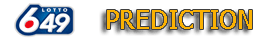# 649 Calculation Prediction

##Beta 1.9.2019

The theory of this prediction is based on following facts: The number of next draw can be get from the pool of the past draws, not all, but 6 or 8 draws.

So that 5 to 6 numbers of the next draw will be from the about 30 number pool. The hit ratio is increased.

Analysis draws, until 4139draw, offset 0~20draws,
past draws. Compare with the target draw.

 Analysis 14 draws, until 4139. Offset 0 draws, past 6 draws 43÷385=0.11168831168831 Go Analysis 14 draws, until 4139. Offset 1 draws, past 6 draws 48÷383=0.12532637075718 Go Analysis 14 draws, until 4139. Offset 2 draws, past 6 draws 46÷384=0.11979166666667 Go Analysis 14 draws, until 4139. Offset 3 draws, past 6 draws 42÷382=0.10994764397906 Go Analysis 14 draws, until 4139. Offset 4 draws, past 6 draws 42÷383=0.10966057441253 Go Analysis 14 draws, until 4139. Offset 5 draws, past 6 draws 44÷385=0.11428571428571 Go Analysis 14 draws, until 4139. Offset 6 draws, past 6 draws 44÷387=0.11369509043928 Go Analysis 14 draws, until 4139. Offset 7 draws, past 6 draws 44÷385=0.11428571428571 Go Analysis 14 draws, until 4139. Offset 8 draws, past 6 draws 46÷383=0.1201044386423 Go Analysis 14 draws, until 4139. Offset 9 draws, past 6 draws 44÷382=0.1151832460733 Go Analysis 14 draws, until 4139. Offset 10 draws, past 6 draws 46÷380=0.12105263157895 Go Analysis 14 draws, until 4139. Offset 11 draws, past 6 draws 47÷378=0.12433862433862 Go Analysis 14 draws, until 4139. Offset 12 draws, past 6 draws 43÷378=0.11375661375661 Go Analysis 14 draws, until 4139. Offset 13 draws, past 6 draws 43÷378=0.11375661375661 Go Analysis 14 draws, until 4139. Offset 14 draws, past 6 draws 40÷379=0.10554089709763 Go Analysis 14 draws, until 4139. Offset 15 draws, past 6 draws 40÷378=0.10582010582011 Go Analysis 14 draws, until 4139. Offset 16 draws, past 6 draws 40÷380=0.10526315789474 Go Analysis 14 draws, until 4139. Offset 17 draws, past 6 draws 40÷382=0.10471204188482 Go Analysis 14 draws, until 4139. Offset 18 draws, past 6 draws 44÷387=0.11369509043928 Go Analysis 14 draws, until 4139. Offset 19 draws, past 6 draws 44÷390=0.11282051282051 Go Analysis 14 draws, until 4139. Offset 20 draws, past 6 draws 48÷390=0.12307692307692 Go